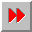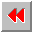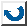EMA-XPS Online

DENOMINATOR

```DENOMINATOR
===========

syntax: (denominator <ratio>)

==> <denominator>

This function extracts the denominator from a ratio.
The ratio has to be of type integer or ratio. If
the number is an integer, then the denominator is 1.

example:       >(denominator 2/3)
3

```EMA-XPS Online